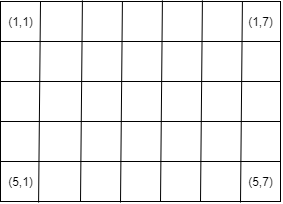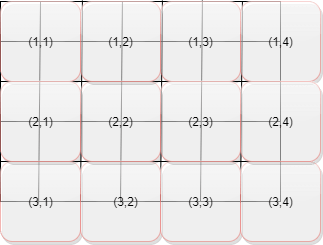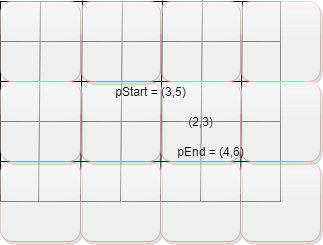# blocksub2sub

Convert block subscripts to pixel subscripts

## Syntax

``[pStart,pEnd] = blocksub2sub(bim,blocksub)``
``[pStart,pEnd] = blocksub2sub(bim,blocksub,'Level',L)``

## Description

example

````[pStart,pEnd] = blocksub2sub(bim,blocksub)` converts a block subscript to pixel subscripts. `pStart` is the pixel subscripts of the first pixel in the block. `pEnd` is the pixel subscripts of the last pixel in the block. Note: For partial blocks `pEnd` represents the last valid pixel subscript in the image.```
````[pStart,pEnd] = blocksub2sub(bim,blocksub,'Level',L)` additionally specifies the resolution level to use in a multiresolution image. `Level` defaults to `1`.```

## Examples

collapse all

Create a small sample image as a 5-by-7 matrix of zeros. Here is an illustration of the small sample image, with the pixel coordinates of the four corners provided.Create a blocked image from the sample image, specifying a 2-by-2 block size. To create this blocked image, use `blockedImage` in write mode.

`bim = blockedImage([],[5 7],[2 2],uint8(0),"Mode",'w');`

Here is an illustration of the blocked image overlaid on the original image. It is divided into 2-by-2 blocks. In the diagram, each block contains its block coordinates.Convert block subscripts into pixel subscripts by using the `blocksub2sub` function. By default, if the image is a multiresolution image, `blocksub2sub` uses pixel coordinates from coarsest level, although you can specify any level. Since the sample image has only one resolution level, `blocksub2sub` converts level 1.

`[pstart,pend] = blocksub2sub(bim,[2 3])`
```pstart = 1×2 3 5 ```
```pend = 1×2 4 6 ```

This illustration show the block coordinate `[2 3]` converted to pixel coordinates.## Input Arguments

collapse all

Blocked image, specified as a `blockedImage` object.

Block subscripts, specified as a numeric array of positive integers.

Example: `[2 3]`

## Output Arguments

collapse all

Subscript of first pixel in the specified block, returned as a numeric array.

Subscript of last pixel in the specified block, returned as a numeric array.

## Version History

Introduced in R2021a